# Area of shape + circular sector - math problems

1. FlakesA circle was described on the square, and a semicircle above each side of the square was described. This created 4 "flakes". Which is bigger: the content of the central square or the content of four chips?
2. Three segments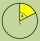The circle is divided into 3 segments. Segment A occupies 1/4 of the area, segment B occupies 1/3 of the area. What part is occupied by section C? In what proportion are areas A: B: C?
3. Quarter of a circleCalculate the circumference of a quarter circle if its content is S = 314 cm2.
4. Irrigation sprinklerThe irrigation sprinkler can twist with an angle of 320° and has a reach of 12 meters. Which area can irrigate?
5. Cylinder horizontallyThe cylinder with a diameter of 3 m and a height/length of 15 m is laid horizontally. Water is poured into it, reaching a height of 60 cm below the axis of the cylinder. How many hectoliters of water is in the cylinder?
6. Circular segmentCalculate the area S of the circular segment and the length of the circular arc l. The height of the circular segment is 2 cm and the angle α = 60°. Help formula: S = 1/2 r2. (Β-sinβ)
7. Equilateral triangle v3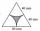Calculate the content of the colored gray part. Equilateral triangle has side length 8 cm. Arc centers are the vertices of a triangle.
8. Math heart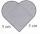Stylized heart shape created from a square with side 5 cm and two semicircles over his sides. Calculate the content area and its circumference.
9. Circular poolThe base of the pool is a circle with a radius r = 10 m, excluding a circular segment that determines the chord length 10 meters. The pool depth is h = 2m. How many hectoliters of water can fit into the pool?
10. Circle sectorCircular sector with a central angle 80 ° has area 1257 cm2. Calculate its radius r.
11. Field with vegetables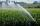Field planted with vegetables has shape of a rectangular isosceles triangle with leg length of 24 m. At the vertices of the triangle are positioned rotating sprinklers with a range of 12 m. How much of the field sprinkler doesn't irrigated?
12. Goat and circlesWhat is the radius of a circle centered on the other circle and the intersection of the two circles is equal to half the area of the first circle? This task is the mathematical expression of the role of agriculture. The farmer has circular land on which
13. Park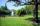In the newly built park will be permanently placed a rotating sprayer irrigation of lawns. Determine the largest radius of the circle which can irrigate by sprayer P so not to spray park visitors on line AB. Distance AB = 55 m, AP = 36 m and BP = 28 m.
14. Circle arcCalculate the area of the circular arc in m2 where the diameter is 290 dm and a central angle is 135°. Result round to three decimal places.Calculate the radius of the quadrant, which area is equal to area of circle with radius r = 15 cm.
16. Arc and segmentCalculate the length of circular arc l, area of the circular arc S1 and area of circular segment S2. Radius of the circle is 11 and corresponding angle is ?.
17. Circle and angleWhat is the length of the arc of a circle with radius r = 207 mm with cental angle 5.33 rad?
18. Circle arcCircle segment has a circumference of 135.26 dm and 2096.58 dm2 area. Calculate the radius of the circle and size of central angle.

Do you have an interesting mathematical word problem that you can't solve it? Submit math problem, and we can try to solve it.

We will send a solution to your e-mail address. Solved examples are also published here. Please enter the e-mail correctly and check whether you don't have a full mailbox.

Please do not submit problems from current active competitions such as Mathematical Olympiad, correspondence seminars etc...